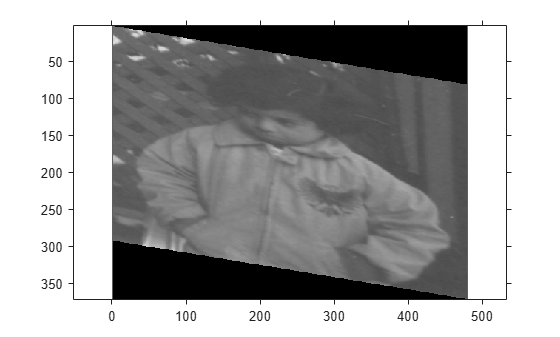Documentation

affine2d

2-D affine geometric transformation

Description

An affine2d object stores information about a 2-D affine geometric transformation and enables forward and inverse transformations.

Creation

You can create an affine2d object using the following methods:

• imregtform — Estimates a geometric transformation that maps a moving image to a fixed image using similarity optimization

• imregcorr — Estimates a geometric transformation that maps a moving image to a fixed image using phase correlation

• fitgeotrans — Estimates a geometric transformation that maps pairs of control points between two images

• randomAffine2d — Creates a randomized 2-D affine transformation

• The affine2d function described here

Description

tform = affine2d creates an affine2d object with default property settings that correspond to the identity transformation.

example

tform = affine2d(A) sets the property T with a valid affine transformation defined by nonsingular matrix A.

Properties

expand all

Forward 2-D affine transformation, specified as a nonsingular 3-by-3 numeric matrix.

The matrix T uses the convention:

[x y 1] = [u v 1] * T

where T has the form:

[a b 0;
c d 0;
e f 1];

The default of T is the identity transformation.

Data Types: double | single

Dimensionality of the geometric transformation for both input and output points, specified as the value 2.

Object Functions

 invert Invert geometric transformation isRigid Determine if transformation is rigid transformation isSimilarity Determine if transformation is similarity transformation isTranslation Determine if transformation is pure translation outputLimits Find output spatial limits given input spatial limits transformPointsForward Apply forward geometric transformation transformPointsInverse Apply inverse geometric transformation

Examples

collapse all

Create an affine2d object that defines a 30 degree rotation in the counterclockwise direction around the origin.

theta = 30;
tform = affine2d([cosd(theta) sind(theta) 0;...
-sind(theta) cosd(theta) 0; 0 0 1])
tform =
affine2d with properties:

Dimensionality: 2
T: [3x3 double]

Apply the forward geometric transformation to a point (10,0).

[x,y] = transformPointsForward(tform,10,0)
x = 8.6603
y = 5

Validate the transformation by plotting the original point (in blue) and the transformed point (in red).

figure
plot(10,0,'bo',x,y,'ro')
axis([0 12 0 12]); axis square;Read an image into the workspace.

Create an affine2d object that defines an affine geometric transformation. This example combines vertical shear and horizontal stretch.

tform = affine2d([2 0.33 0; 0 1 0; 0 0 1])
tform =
affine2d with properties:

Dimensionality: 2
T: [3x3 double]

Apply the geometric transformation to the image using imwarp.

B = imwarp(A,tform);

Display the resulting image.

figure
imshow(B);
axis on equal;